Next: Convective Instability Up: Gravitational Instability of Thin Previous: Gravitational Instability of Thin   Contents

## Rotating Disk

In the case of a rotating disk with an angular rotation speed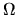, basic equations are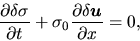(2.66)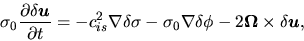(2.67)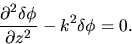(2.68)

Equation (2.68) gives a solution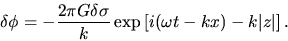(2.69)

Choosing a direction in which the wavenumber vector can be expressed as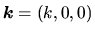, we can reduce equations (2.66) and (2.67) to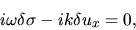(2.70)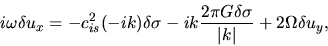(2.71)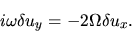(2.72)

These three equations together with equation (2.69) bring us a dispersion relation as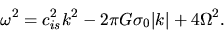(2.73)

Comparing with equation(2.65), this indicates rotation works to stabilize the system.

Equation (2.73) is rewritten as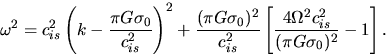(2.74)

Defining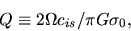(2.75)

we can see that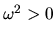for all wavenumbers if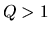and that if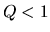for some range of wavenumber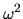becomes negative. A rotating disk withis unstable for some range of wavenumber. This number is called Toomre's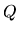. This is useful to see whether a galactic disk is stable or not. For the galactic disk, Toomre'smust be modified as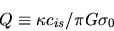(2.76)

where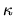represents the epicyclic frequency as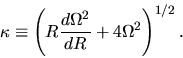(2.77)

See section 3.4 for the galactic disk.Next: Convective Instability Up: Gravitational Instability of Thin Previous: Gravitational Instability of Thin   Contents
Kohji Tomisaka 2007-07-08